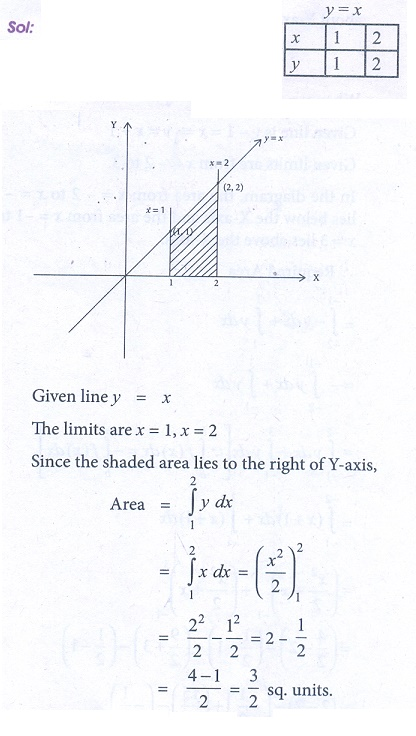Home | | Business Maths 12th Std | Exercise 3.1: The area of the region bounded by the curves

# Exercise 3.1: The area of the region bounded by the curves

Book back answers and solution for Exercise questions - Maths: Integral Calculus: The area of the region bounded by the curves: Geometrical Interpretation of Definite Integral as Area under a curve: Problem Questions with Answer, Solution

Exercise 3.1

1. Using Integration, find the area of the region bounded the line 2 y + x = 8, the x axis and the lines x = 2, x = 4.2. Find the area bounded by the lines y ŌłÆ 2x ŌłÆ 4 = 0, y = 1, y = 3 and the y-axis3. Calculate the area bounded by the parabola y2 = 4ax and its latusrectum.4. Find the area bounded by the line y = x, the x-axis and the ordinates x = 1, x = 2.5. Using integration, find the area of the region bounded by the line y ŌłÆ 1 = x ,the x axis and the ordinates x = ŌĆō2, x = 3.6. Find the area of the region lying in the first quadrant bounded by the region = 4x2 , x = 0, y = 0 and y = 4.7. Find the area bounded by the curve y = x2 and the line y = 4